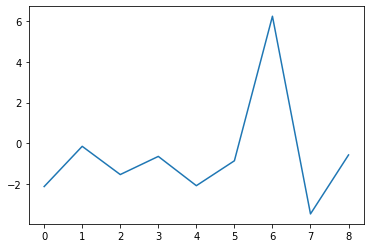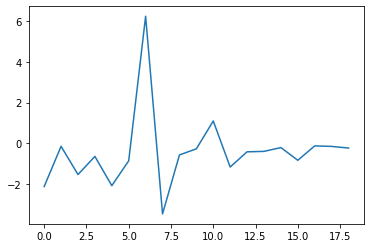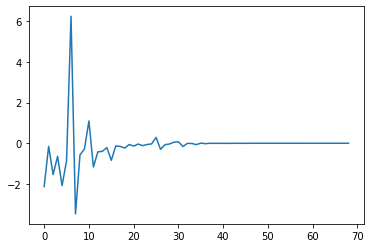Due to the way it mixes several – relatively – simple concepts, Bayesian optimization (BO) is one of the most elegant mathematical tool I’ve encountered so far. In this post, I introduce GPopt, a tool for BO that I implemented in Python (no technical docs yet, but coming soon). The examples of GPopt’s use showcased here are based on Gaussian Processes (GP) and Expected Improvement (EI): what does that mean?

GPs are Bayesian statistical/machine learning (ML) models which create a distribution on functions, and especially on black-box functions. If we let $$f$$ be the black-box – and expensive-to-evaluate – function whose minimum is searched, a GP is firstly adjusted (in a supervised learning way) to a small set of points at which $$f$$ is evaluated. This small set of points is the initial design. Then the GP, thanks to its probabilistic nature, will exploit its knowledge of previous points at which $$f$$ has already been evaluated, to explore new points: potential minimas maximizing an EI criterion. It’s a reinforcement learning question!

For more details on Bayesian optimization applied to hyperparameters calibration in ML, you can read Chapter 6 of this document. In this post, a Branin (2D) and a Hartmann (3D) functions will be used as examples of objective functions $$f$$, and Matérn 5/2 is the GP’s covariance.

Installing and importing the packages:

!pip install GPopt

!pip install matplotlib==3.1.3

import GPopt as gp
import time
import sys
import numpy as np
import matplotlib.pyplot as plt
from os import chdir
from scipy.optimize import minimize


The objective function to be minimized:

# branin function
def branin(x):
x1 = x
x2 = x
term1 = (x2 - (5.1*x1**2)/(4*np.pi**2) + (5*x1)/np.pi - 6)**2
term2 = 10*(1-1/(8*np.pi))*np.cos(x1)
return (term1 + term2 + 10)

# A local minimum for verification
print("\n")
res = minimize(branin, x0=[0, 0], method='Nelder-Mead', tol=1e-6)
print(res.x)
print(branin(res.x))

[3.14159271 2.27500017]
0.397887357729795


Firstly, I demonstrate the convergence of the optimizer towards a minimum (and show that the optimizer can pick up the procedure where it left):

# n_init is the number of points in the initial design
# n_iter is the number of iterations of the optimizer
gp_opt = gp.GPOpt(objective_func=branin,
lower_bound = np.array([-5, 0]),
upper_bound = np.array([10, 15]),
n_init=10, n_iter=10)
gp_opt.optimize(verbose=1)


Plotting the changes in expected improvement as a function of the number of iterations.

print(gp_opt.x_min) # current minimum
print(gp_opt.y_min) # current minimum
plt.plot(np.diff(gp_opt.max_ei))

[9.31152344 1.68457031]
0.9445903336427559Adding more iterations to the optimizer:

gp_opt.optimize(verbose=1, n_more_iter=10)


...Done.

Optimization loop...

10/10 [██████████████████████████████] - 2s 186ms/step

(array([3.22692871, 2.63122559]), 0.6107733232129569)


Plotting the changes in expected improvement as a function of the number of iterations (again).

print(gp_opt.x_min) # current minimum
print(gp_opt.y_min) # current minimum
plt.plot(np.diff(gp_opt.max_ei))

[3.22692871 2.63122559]
0.6107733232129569Adding more iterations to the optimizer (again):

gp_opt.optimize(verbose=1, n_more_iter=80)


Plotting the changes in expected improvement as a function of the number of iterations (again).

print(gp_opt.x_min) # current minimum
print(gp_opt.y_min) # current minimum
plt.plot(np.diff(gp_opt.max_ei))

[9.44061279 2.48199463]
0.3991320518189241The 3 previous graphs suggest the possibility of stopping the optimizer earlier, when the algorithm is not improving on previous points’ results anymore:

# # early stopping
# abs_tol is the parameter that controls early stopping

gp_opt2 = gp.GPOpt(objective_func=branin,
lower_bound = np.array([-5, 0]),
upper_bound = np.array([10, 15]),
n_init=10, n_iter=190)
gp_opt2.optimize(verbose=2, abs_tol=1e-4)
print("\n")


We can observe that only 58 iterations are necessary when abs_tol = 1e-4

print(gp_opt2.n_iter)
print(gp_opt2.x_min)
print(gp_opt2.y_min)

58
[9.44061279 2.48199463]
0.3991320518189241


Illustrating convergence:

plt.plot(gp_opt2.max_ei)We notice that in this example, GPopt falls into a local minimum but is very close to the previous minimum found with gradient-free optimizer (Nelder-Mead). The opposite situation can occur too:

# [0, 1]^3
def hartmann3(xx):

alpha = np.array([1.0, 1.2, 3.0, 3.2])

A = np.array([3.0, 10, 30,
0.1, 10, 35,
3.0, 10, 30,
0.1, 10, 35]).reshape(4, 3)

P = 1e-4 * np.array([3689, 1170, 2673,
4699, 4387, 7470,
1091, 8732, 5547,
381, 5743, 8828]).reshape(4, 3)

xxmat = np.tile(xx,4).reshape(4, 3)

inner = np.sum(A*(xxmat-P)**2, axis = 1)
outer = np.sum(alpha * np.exp(-inner))

return(-outer)

# Fails, but may work with multiple restarts
print("\n")
res = minimize(hartmann3, x0=[0, 0, 0], method='Nelder-Mead', tol=1e-6)
print(res.x)
print(hartmann3(res.x))

[0.36872308 0.11756145 0.26757363]
-1.00081686355956

# hartmann 3D
gp_opt4 = gp.GPOpt(objective_func=hartmann3,
lower_bound = np.repeat(0, 3),
upper_bound = np.repeat(1, 3),
n_init=20, n_iter=280)
gp_opt4.optimize(verbose=2, abs_tol=1e-4)

print(gp_opt4.n_iter)
print(gp_opt4.x_min)
print(gp_opt4.y_min)
print("\n")

51
[0.07220459 0.55792236 0.85662842]
-3.8600590626769904


The question is, in the case of BO applied to ML cross-validation, do we really want to find the global minimum of the objective function?